or# RD Sharma Class 12 and Chapter Differentiation Maths Solutions

## Maths Class 12 and Chapter Differentiation RD Sharma SolutionsFilter
Filters :
CLASSES
•
•
•
•
•
•
•
CHAPTERS
•
• 24 More

We all cherish so many memories in our life but out of all the memories that we lived once, school times are the best. Whether you accept it or not, the friends that we made, the memories that we together created, and the marks we obtained altogether shape our career and make us what we are today.

Where it is true that school times are counted and remembered lifelong, the resources that we studied from on the other side also plays a vital role in our career.

And that's why it is very important to always go with a set of books that contains a detailed explanation of each topic and multiple questions to practice. After all, your career and future happiness majorly based on the success you grab in school.

If you're a student reading this right now, you must understand the importance of R.D. Sharma books in your school phase. These help books are the best available resource for students who want to excel in CBSE exams and competitive exams.

Not to mention but students will also be able to find accurate solutions of miscellaneous exercises given at the end of each chapter in NCERT.

Because according to experienced teachers and council of CBSE board, most of the questions come directly from the exercises given in the NCERT books. And that's why it is important to have precise answers with step by step solutions.

## INTRODUCTION:

The rate of change of a quantity y with respect to quantity x in a given equation is called the derivative or differential coefficient of y with respect to x.

## Differentiation of a Function

Let f(x) be a function differentiable in an interval [a, b]. At every point of the interval, the derivative of the function occurs finitely and is unique. Henceforth, we may define a new function g: [a, b] R, such that, x [a, b], g(x) = f'(x).
This new function is said to be differentiation (differential coefficient) of the function f(x) with respect to x and it is denoted by df(x) / d(x) or Df(x) or f'(x).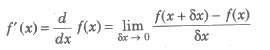### Standard Differentiations

1. d / d(x) (xn) = nxn 1, x R, n R
2. d / d(x) (k) = 0, where k is constant.

3. d / d(x) (ex) = ex
4. d / d(x) (ax) = ax loge a > 0, a ? 1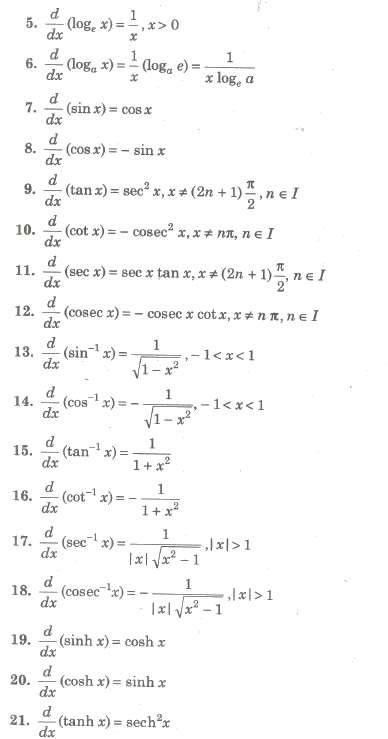### Fundamental Rules for Differentiation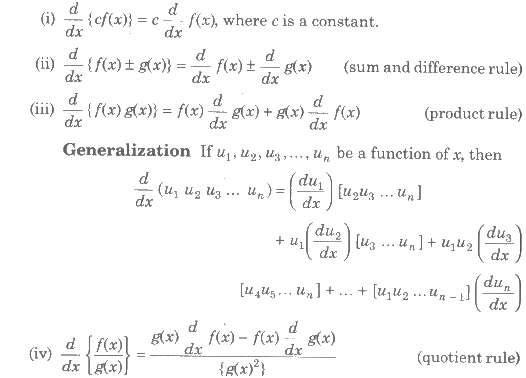(v) if d / d(x) f(x) = (x), then d / d(x) f(ax + b) = a ?(ax + b)
(vi) Differentiation of a constant function is always zero i.e., d / d(x) (c) = 0.

## Geometrically Meaning of Derivative at a Point

The Geometrically derivative of a function at a point x = c is, the slope of the tangent to the curve y = f(x) at the point {c, f(c)}.
Slope of tangent= lim
x - c f(x) f(c) / x c = {df(x) / d(x)} x = c or f (c).

## Different Types of Differentiable Function

### Differentiation of Composite Function (Chain Rule)

If f and g are differentiable functions in their domain, then (fog) is also differentiable and
(fog)(x) = f {g(x)} g (x)
More easily, if y = f(u) and u = g(x), then dy / dx = dy / du * du / dx.
If y is a function of u, u is a function of v and v is a function of x. Then, dy / dx = dy / du * du / dv * dv / dx.

### Differentiation Using Substitution

In order to find differential coefficients of difficult expression involving inverse trigonometric functions then, some substitutions are very supportive, which are listed below.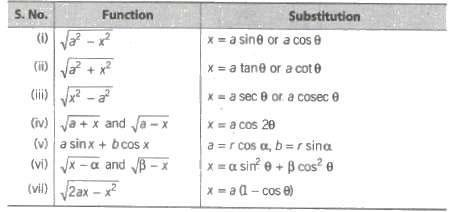### Differentiation of Implicit Functions

If f(x, y) = 0, and differentiate with respect to x and obtain the terms which contain dy / dx at one side and find dy / dx.
Shortcut for Implicit Functions For Implicit function, put d /dx {f(x, y)} = f / x / f / y, where f / x is a partial differential of given function with respect to x and f / y which shows Partial differential of given function with respect to y.

### Differentiation of Parametric Functions

If x = f(t), y = g(t), where t is limit, then dy / dx = (dy / dt) / (dx / dt) = d / dt g(t) / d / dt f(t) = g (t) / f (t)

### Differential Coefficient Using Inverse Trigonometrical Substitutions

Sometimes the given function can be deducted with the help of inverse Trigonometrical substitution and then to find the differential coefficient is very easy.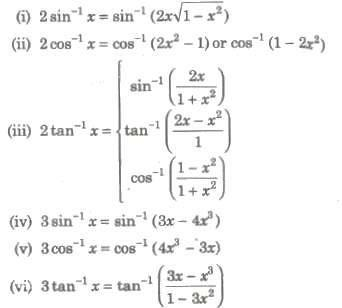## Logarithmic Differentiation Function

(i) If a function is the product and quotient of functions such as y = f1(x) f2(x) f3(x) / g1(x) g2(x) g3(x) , we first take algorithm and then differentiate.
(ii) If a function is in the form of exponent of a function concluded another function such as [f(x)]
g(x) , we first take logarithm and then differentiate.

## Differentiation of a Function with Respect to Another Function:

Suppose y = f(x) and z = g(x), then the differentiation of y with respect to z came as dy / dz = dy / dx / dz / dx = f (x) / g(x)

## Successive Differentiations

If the function y = f(x) be differentiated with respect to x, then the result dy / dx or f(x), so obtained is a function of x (maybe a constant).
Therefore, dy / dx can again be differentiated with respect to x.
The differential coefficient of dy / dx with respect to x is written as d /dx (dy / dx) = d
2y / dx2 or f(x). Again, the differential coefficient of d2y / dx2 with respect to x is written as d / dx (d2y / dx2) = d3y / dx3 or f'(x)
Here, dy / dx, d
2y / dx2, d3y / dx3, are respectively known as first, second, third, order differential coefficients of y with respect to x. These alternatively denoted by f (x), f (x), f
(x), or y
1, y2, y3., respectively.
Note dy / dx = (dy / d) / (dx / d) but d2y / dx2(d2y / d?2) / (d2x / d?2)

#### Leibnitz Theorem

If u and v are functions of x and their nth derivative exist, then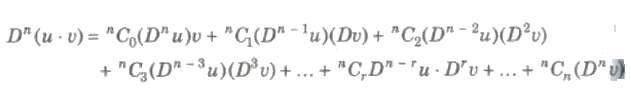## nth Derivative of Some Functions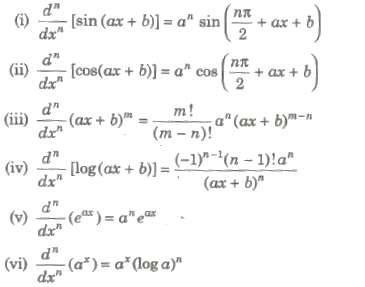### Derivatives of Special Types of Functions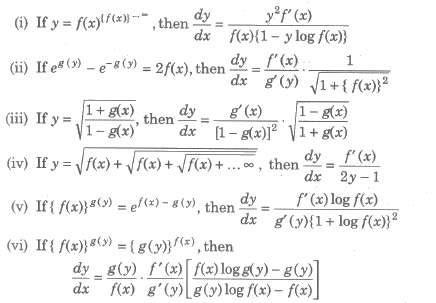(vii) Differentiation of a Determinant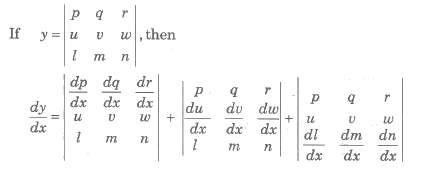##### Partial Differentiation

The partial differential coefficient of f (x, y) with respect to x is the differential coefficient of f (x, y) where y is considered as a constant, then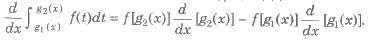## IMPORTANCE OF RD SHARMA CLASS 12TH Math

1. The twelfth standard Mathematics RD Sharma gives understudies right ideas from the base and helps to comprehend the themes in a wide way.

2. The competitors who continually alluded RD Sharma help guides for discharge the course to advance since it gives fundamental thoughts as opposed to sounding the formula-based request direct.

3. We all understand that maths isn't composing type subject that we can learn Instead, it is a subject that requires perfect learning of plans and practicing the request as much as a competitor bore licenses.

4. RD Sharma 12th class maths books have different practice questions and exercises to gauge to even more promptly comprehend the essential thought of request and how to settle it.

5. Abstracted and assembled via preparing section masters, these books are sorted out as indicated by the present test structures. The new arrival of the book contains all the continuous changes and are twofold checked by experienced educators.

6. As the book contains both, appreciated and unsolved request, the competitors can find the plans of such practice rehearses online moreover. Thus, RD Sharma books are gathered charmingly and bolstered with online plans.